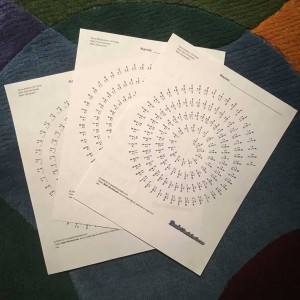Math Worksheets

# Check Out These Addition, Subtraction and Division Spiral Worksheets!Were you waiting for more spiral worksheets for the other math facts? Spiral fact worksheets for addition, subtraction and division have joined the multiplication worksheets posted earlier, and you can find all of them at the links below! Spiral Addition Math Fact Worksheets Spiral Subtraction Math Fact Worksheets Spiral Multiplication Math Fact Worksheets Spiral Division Math Fact Worksheets